# Introduction

Introduced in the Editor v2.0.0 as a POC, the `Graph Editor` is now live starting from the Editor v3.2.0. As all other tools available in the Editor, the `Graph Editor` is available over the main toolbar in `Tools -> Graph Editor`: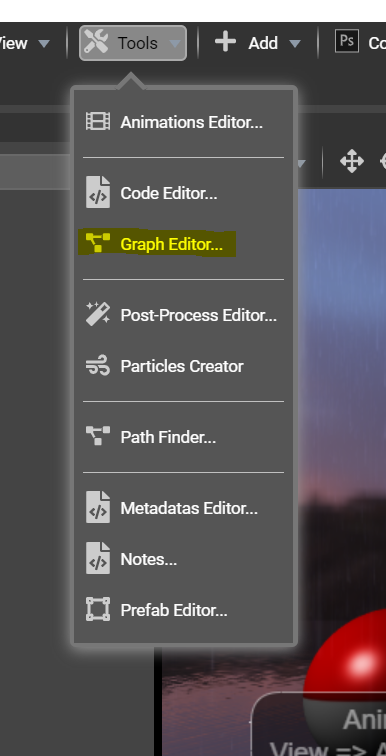# Understanding the tool

As for the Node Material Editor, the `Graph Editor` is used to create custom behaviors for elements in the scene without having to know how to code. The `Graph Editor` tends to evoluate and provide features such as the Unreal Engine Blueprints

The way to attach graphs to elements of the scene is the same as the `Code Editor`:

• Select an element in the scene
• Click `Add New Graph`
• Give it a name
• Add, edit and remove nodes from the graph

All graphs are standalone and can be attached to multiple elements in the scene by clicking `Add` in the list on the left side of the tool.

# Adding nodes by the example

Once a graph has been created and attached to an element of the scene, the goal is to add nodes and connect them to finally create a real algorithm.

Let's have a sphere and try the following example: on the user clicks on the sphere, we want to translate the sphere from x=0,y=0,z=0 to x=2,y=3,z=4.

First, let's add a node. By default, a graph is empty showing an empty canvas: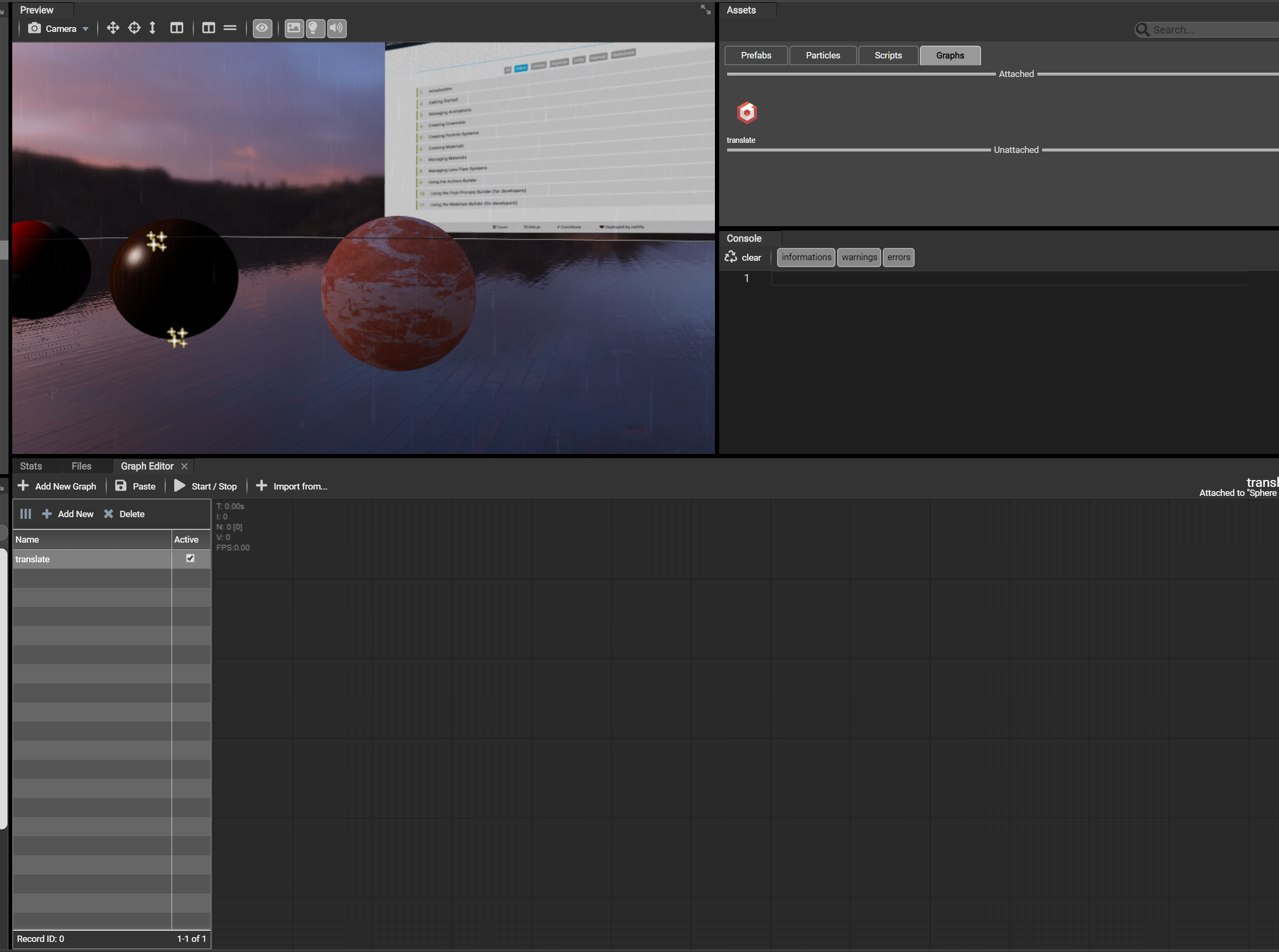To add a new node, just right click on the canvas and a popup will appear to select a node to add. By default, the `search` input is focused and you can research a node by name. Let's search `"PointerDown"` and press `Enter` or double click the item in the list to add in the graph: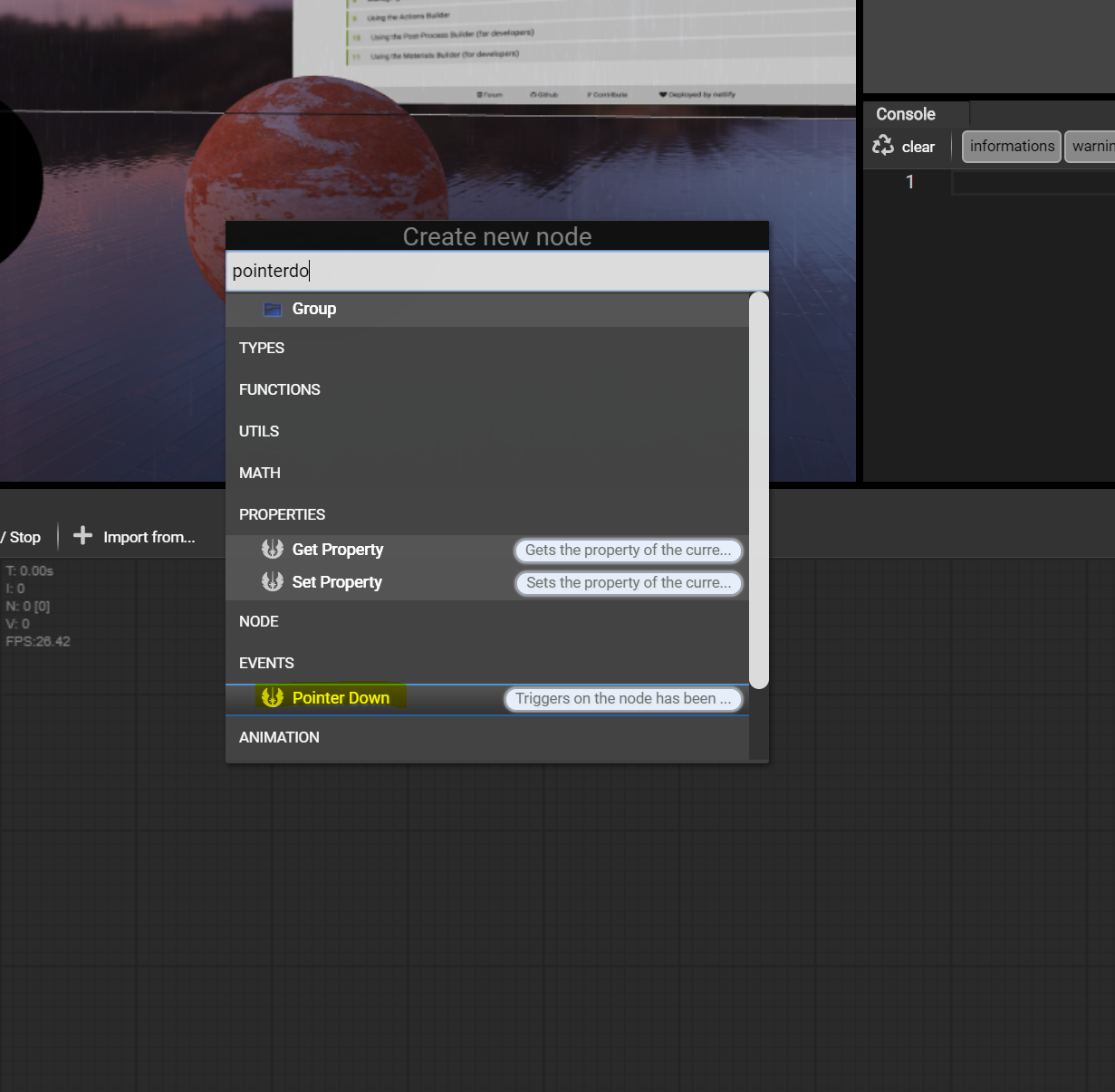The node is now in the graph and shows one output: a rectangle. All inputs/ouputs are identified by their form:

• Rectangle: means `executable`, can send/receive an event. These are called `"triggerable nodes"`.
• Circle: defines an input or output value. These are just receiving or outputing values.

From now, our `PointerDown` node is in the graph and is ready to trigger some nodes. It exists a large list of available nodes and here a particular one will be useful: the `Transform` node. This node will allow to modify the transforms (position, rotation and scale) the current node. Let's right click the graph and search for `"Transform"` and add the node: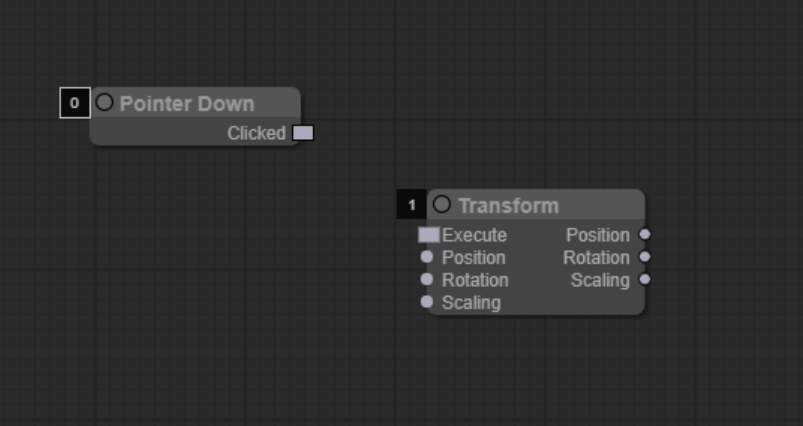As we can see, the `Transform` node is `triggerable` as the first input is a rectangle. All other inputs/outputs are values as their forms are circle. All rectangles/circles on the left side are inputs and all rectangles/circles on the right side are outputs.

To connect nodes, let's drag'n'drop the `PointerDown` node output to the first input of the `Transform` node: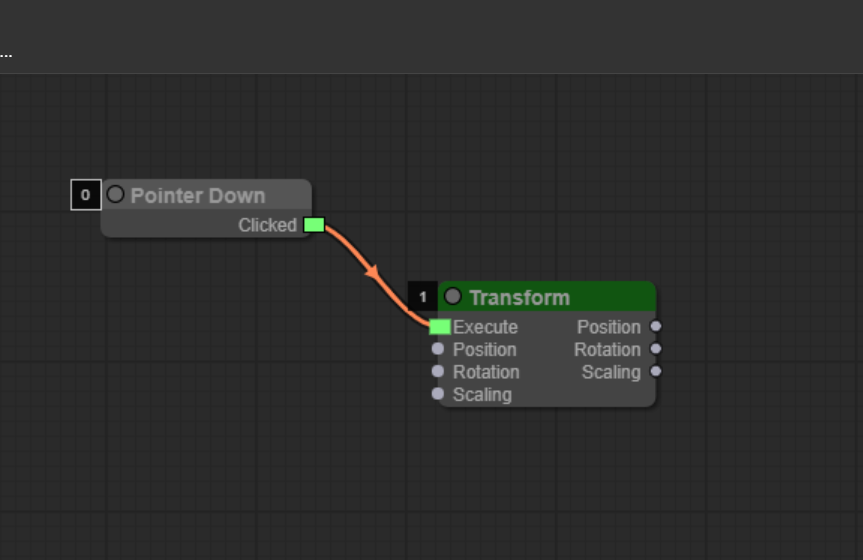Once connected, the top color of the `Transform` node changed and became green. That means the execution time change:

• Grey color means "always executing"
• Green color means "executed on triggered"

In our case, the `Transform` node will be executed ONLY and ONLY when the user clicks on the sphere.

Back to the example, we want the sphere to have a new position x=2,y=3,y=4. That means the input "position" must receive a value to change the transform of the sphere. Let's add a new type of node called `"Type Nodes"`. Type nodes are just nodes that define a type (number, string, vector, color, etc.). In our case, the position is a Vector 3D, so let's add a new Vector3 node: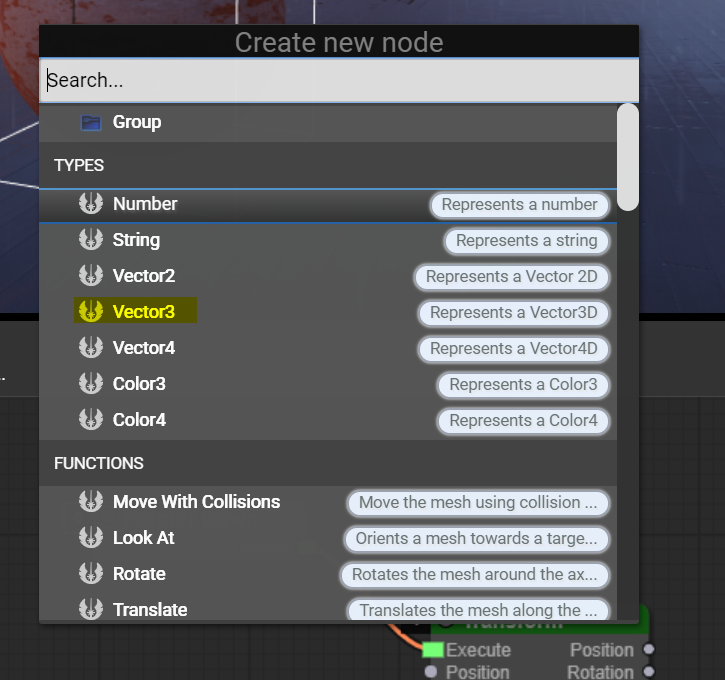Once added, the output `"value"` (which is the vector reference itself) can be connected to the input `"position"` of the `Transform` node: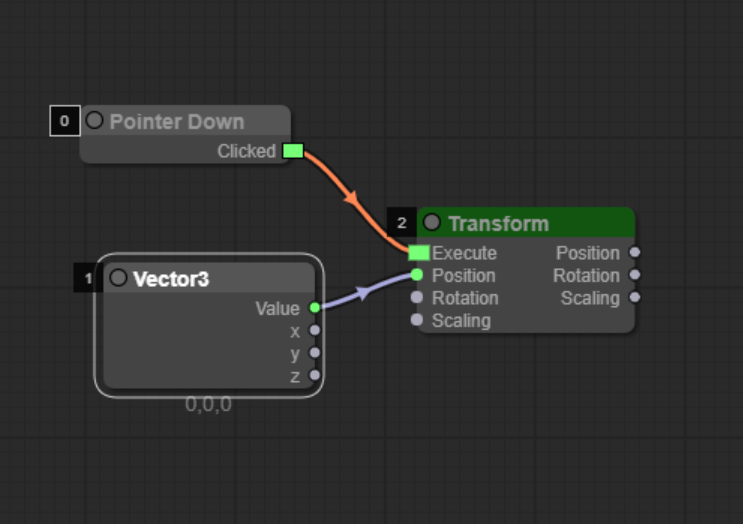Almost all nodes have options and can be customized. This is the case of the `Vector3` node, the values x, y, and z can be modified. So the output of the node can be customized. Let's click the `Vector3` node and go to the Editor's Edition Tools: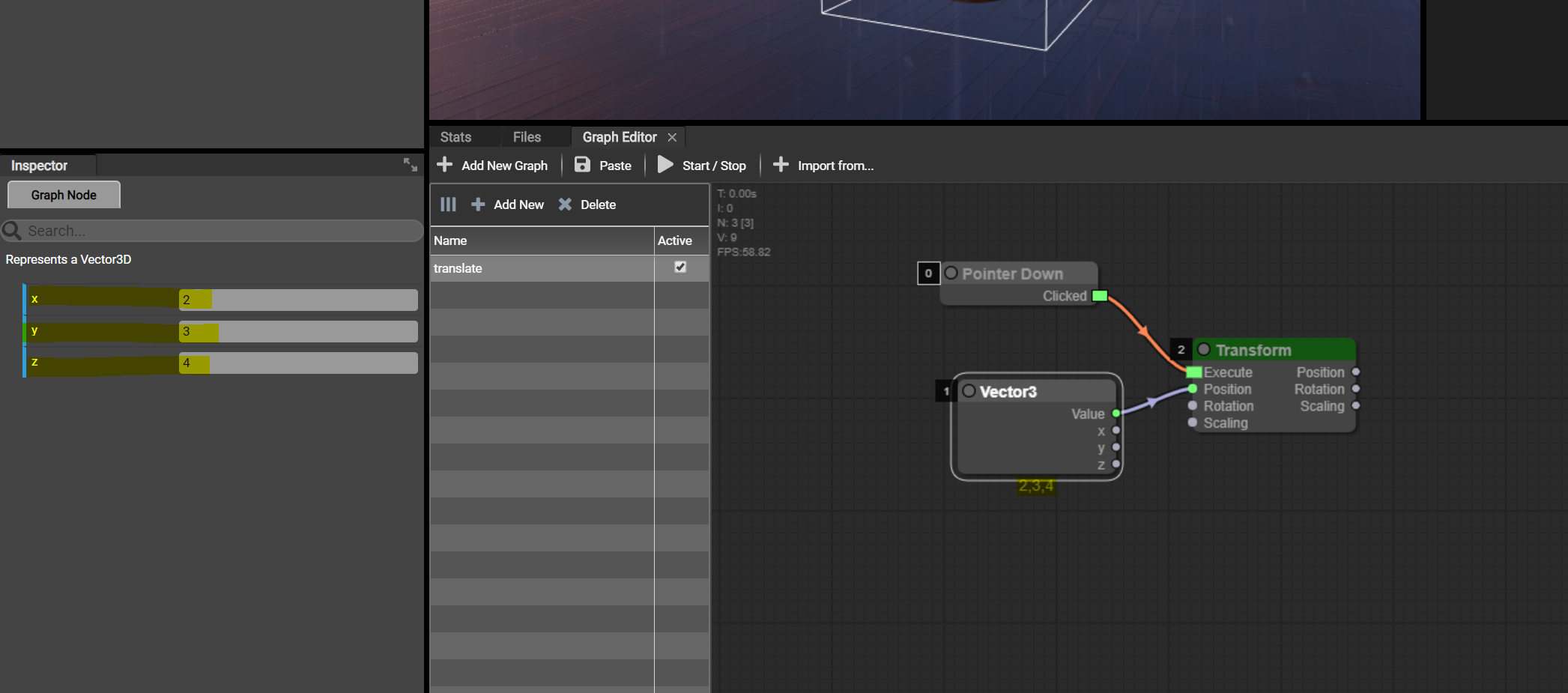The edition tool shows all the customizable properties. Here, the values x, y and z can be customized.

Finally, let's test our graph! To live test a graph, just click the button `Start/Stop` in the Graph Editor an then click the sphere. The connection between the `PointerDown` and `Transform` nodes will be animated to show an activity: the `PointerDown` node triggered the output on the sphere has been clicked and the `Transform` node has be triggered to change the position of the sphere. That's all!

# Using variables

Each graph can have a list of variables. All variables are scoped and available ONLY and ONLY in the graph's context. These are used to store values that support read/write. Variable can be read and written using the `Variable` and are identified by a name, like when writing code.

Variables support the following types:

• String
• Boolean
• Number
• Vector 2D
• Vector 3D
• Vector 4D

To add a variable, just click on the graph and the Editor's Edition Tools will be updated to display a list of available variables (empty by default):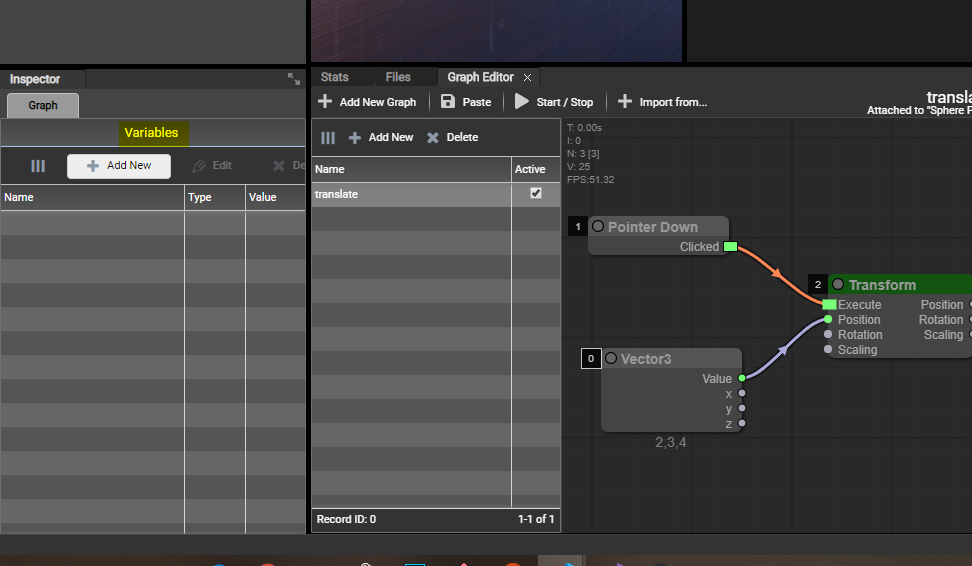Let's modify the example and replace the `Vector3` node by a `Variable` node. To add a variable, just click `Add New` in the variables list, then give it a name and a type. Here, `Vector 3D`: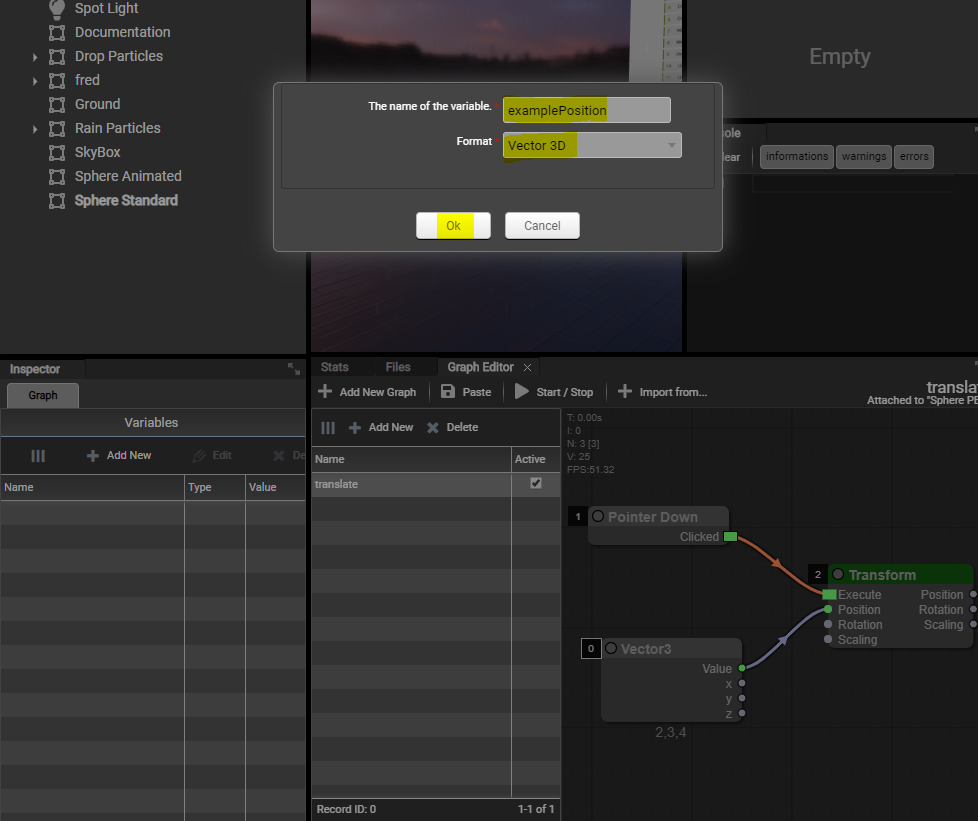Once added, the variable appears in the variables list and can be modified by clicking on the button `Edit`. Let's set the variables values to x=2,y=3,z=4: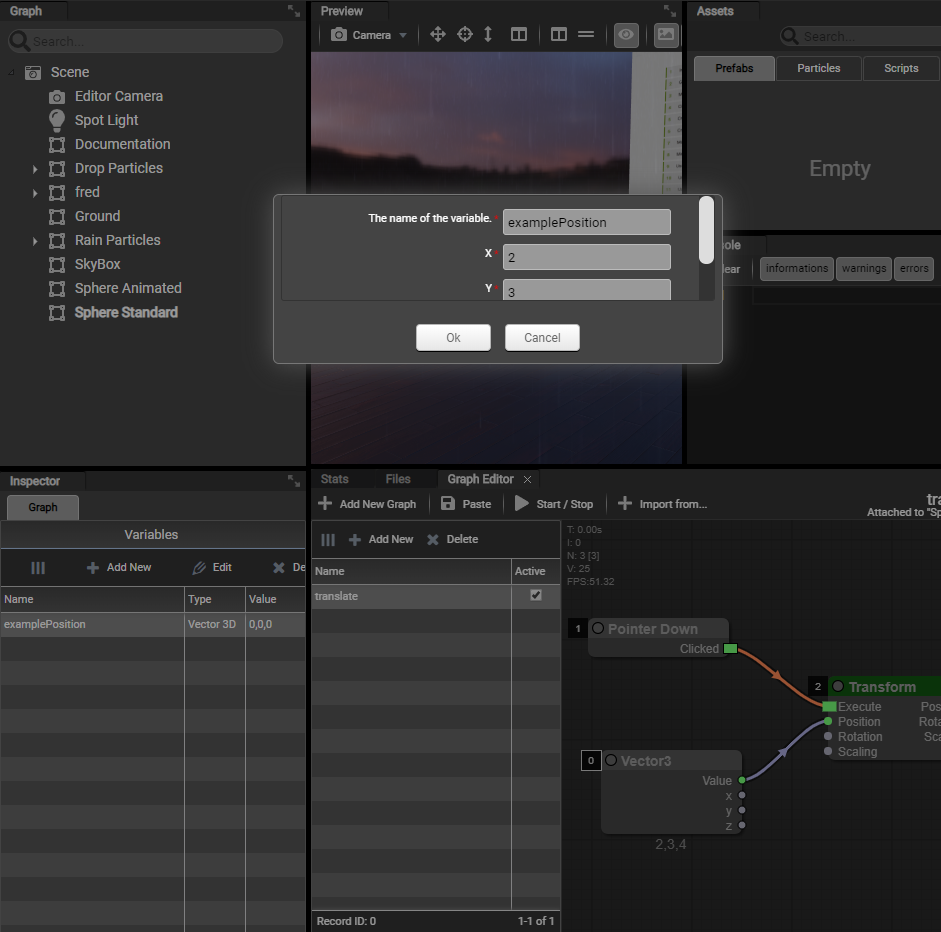The variable is now available to use and is configured. Just add the variable to the graph: right click the canvas and add a new node of type `Variable`. Once added, a combo box is available on the node and can be customized to select the wanted variable: just select your variable:From now, what we have to do is to remove the constant Vector 3D previously added and replace by our variable: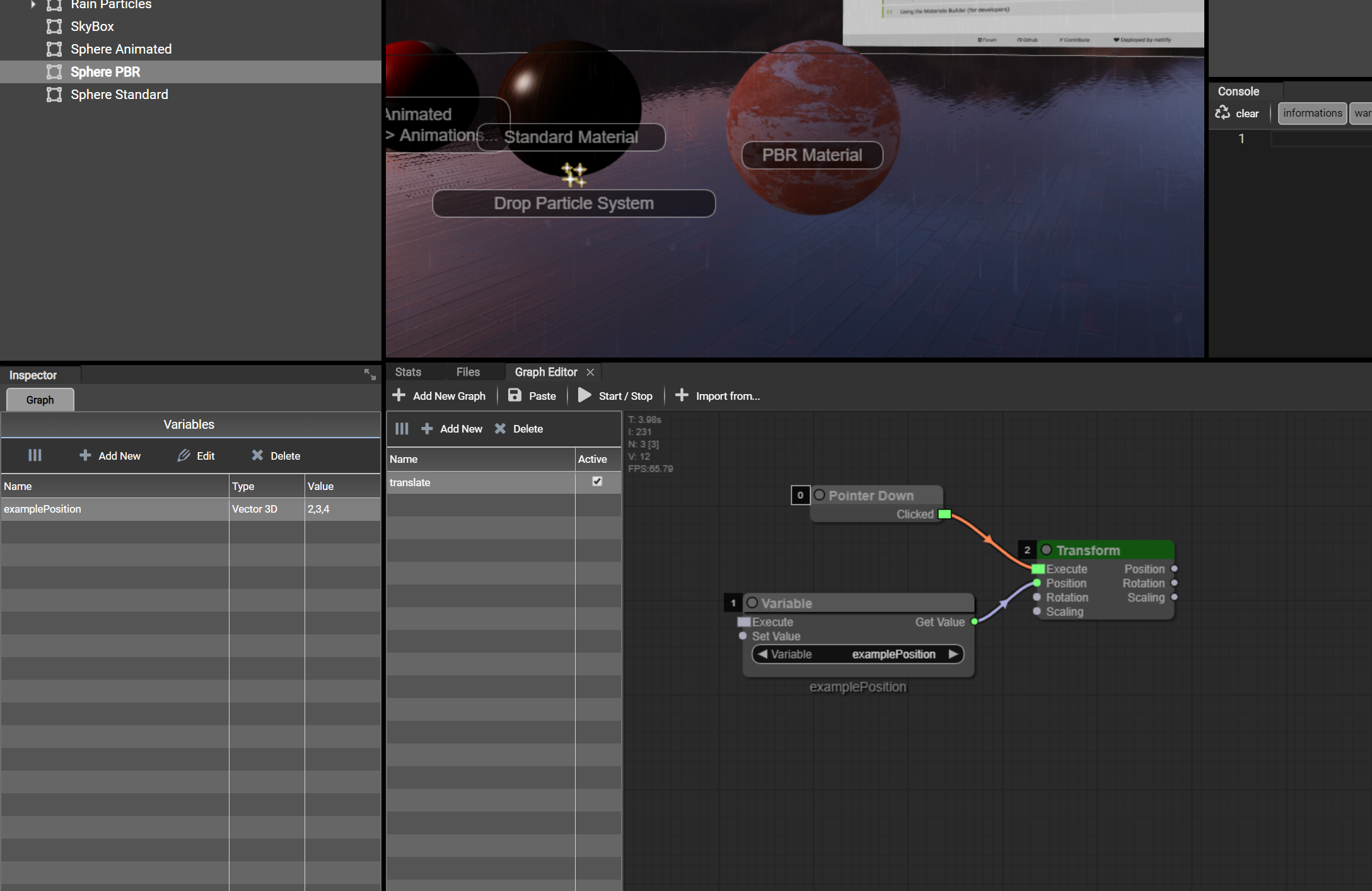Let's take this graph as example to illustrate a more advanced behavior. The goal is to rotate the sphere on the Y axis on the pointer is over the sphere. Then, once the pointer is out, restore the rotation on the Y axis to 0: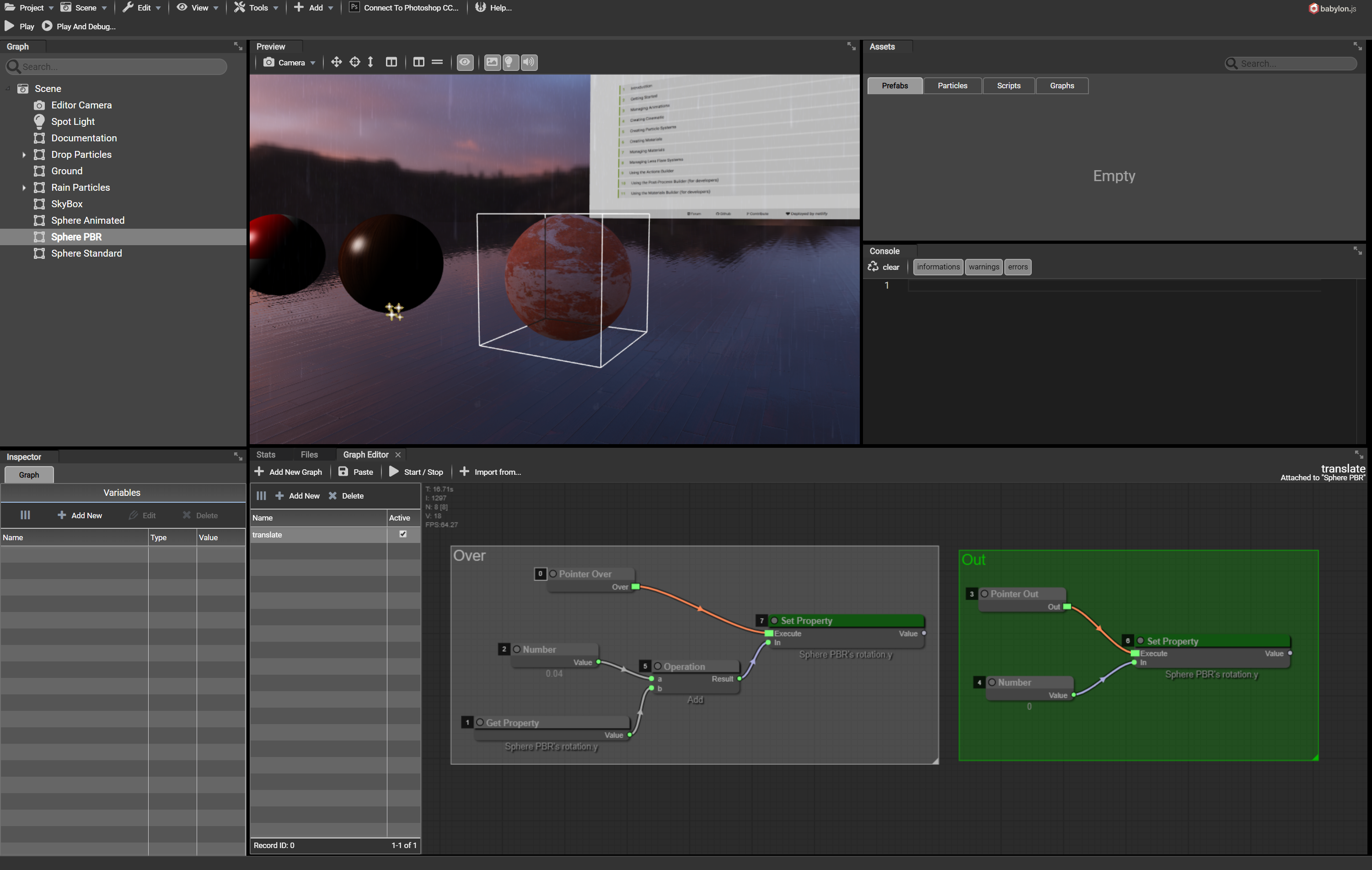Teaching logic can't be done here. So, let's experiment with all other available nodes and create awesome graphs for awesome behaviors in your scene.

# List of all available nodes

• Types
• `Number`: represents a number and outputs its value
• `String`: represents a string and outputs its value
• `Vector2`: represents a Vector 2D and outputs the vector itself, x, and y
• `Vector3`: represents a Vector 2D and outputs the vector itself, x, y and z
• `Vector4`: represents a Vector 2D and outputs the vector itself, x, y, z, and w
• `Color3`: represents a Color 3 (rgb) and outputs the color itself, red, green, and blue
• `Color4`: represents a Color 4 (rgba) and outputs the color itself, red, green, blue and alpha
• Properties: used to manipulate properties of scene elements and variables
• `Get Property`: outputs the property value of the given scene element (see node's properties)
• `Set Property`: sets the given value to the given property of the given scene element (see node's properties)
• `Variable`: adds a variable node available in the graph to output its value and modify its value (see node's properties)
• Functions
• `Move With Collisions`: preforms a translation of the node using the given displacement vector by taking care of the collisions in the scene
• `Look At`: computes the rotation needed for the element to look at the given target point. (yawCor, pitchCor and rollCor are optional)
• `Rotate`: rotates the current element in the given axis at the given amount
• `Translate`: translates the current element in the given axis at the given amount
• `Set Direction`: computes the rotation needed for the element to look at the given direction (`localAxis`). For example Vector3(0, 0, 1) will look forward
• Utils
• `Bypass Type`: takes an input and transforms its type to an "any" type. Allows to connect the output to any other input in the graph by bypassing the type checking. Use with care
• `Time`: outputs the current time in terms of milliseconds or seconds
• `Log`: logs the given message in the console. Useful to help debugging
• `Set Timeout`: creates a new timer and triggers the first output once the specified time has been elapsed. outputs the id of the timeout
• `Clear Timeout`: clears an existing timeout by taking the timeout id as parameter
• `Vector 2D(3D)(4D) to XY(Z)(W)`: takes a vector 2D, 3D or 4D (according to the node) and splits its values to output numbers x, y, z and w
• `Color 3(4) to RGB(A)`: takes a color 3 or 4 (accordiong to the node) and splits its values to output numbers r, g, b and a
• `Vector Merger`: takes numbers as input and ouputs a new vector 2D, 3D or 4D. Vectors 3D and 4D must have their inputs filled
• `Send Script Message`: used while using Code Editor in the same project, sends a message to the given target element in the scene by calling the given method name (see node's properties)
• Math:
• `Scale`: scales the input number of vector using the given scale number (see node's properties)
• `Operation`: performs the given operation (see node's properties) on the given inputs (numbers) and outputs its result. Supported:
• multiply
• subtract
• divide
• `Vector Operation`: performs the given operation (see node's properties) on the given inputs (vectors) and ouputs its result. Supported:
• multiply
• subtract
• divide
• `Fract`: computes the fractional part of the given input (vector)
• `Negate`: negates the given input (number of vector) and ouputs its result
• `Condition`: checks the given inputs and triggers the appropriate output by checking their values (equal, different, etc.)
• `Cosinus`: outputs the value of the cosinus of the given input
• `Sinus`: outputs the value of the sinus of the given input
• `Tangent`: outputs the value of the tangent of the given input
• `Abs`: ouputs the absolute value of the given input
• Node: defines all the available methods/properties for scene nodes
• `Transform`: ouputs all the transform values of the node (position, rotation and scale) and takes them as input too
• `Set Parent`: sets the given parent to the current scene element. Input is of type "mesh" and can be retrieved using the `Get Node By Name` node
• `Get Mesh Direction`: outputs the current direction of the mesh according to its current rotation in the given axis to rotate. Axis (0, 0, 1) will take Z as forward axis
• `Set Mesh Direction`: sets the new direction of the mesh using the given local axis. Axis (0, 0, 1) will take Z as forward axis
• Events: defines all possible events to listen
• `Pointer Down`: triggers the output on the scene element is being touched/clicked
• `Pointer Move`: triggers the output on the mouse moves on the scene element
• `Pointer Up`: triggers the output on the scene element has been clicked
• `Pointer Over`: triggers the output on the mouse is over the scene element
• `Pointer Out`: triggers the output on the mouse is out of the scene element. Will be triggered only and only if the mouse was previously over the scene element
• `Keyboard Down`: triggers the output on the given key (see node's properties) is down
• `Keyboard Up`: triggers the output on the given key (see node's properties) is up
• Animation:
• `Play Animations`: plays the animations of the given target using the given properties (see node's properties)
• `Stop Animations`: stops the animations of the given target (see node's properties)
• `Interpolate Value`: animates the given property of the given target at the given parameters (see node's properties)
• Sound:
• `Play Sound': plays the given sound available in the scene (see node's properties)
• `Pause Sound`: pauses the given sound available in the scene (see node's properties)
• `Stop Sound`: stops the given sound available in the scene (see node's properties)
• Scene:
• `Get Node By Name`: gets a node identified by the given name (see node's properties) and outputs its reference (type "node")
• `Get Mesh By Name`: gets a mesh identified by the given name (see node's properties) and outputs its reference (type "mesh")